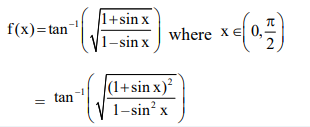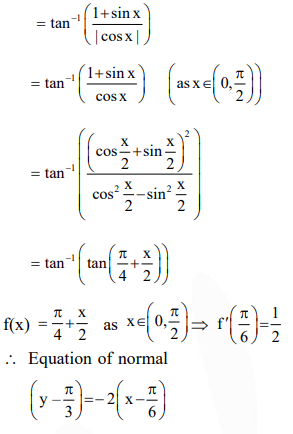# Solve this followingQuestion:

Consider $f(x)=\tan ^{-1}\left(\sqrt{\frac{1+\sin x}{1-\sin x}}\right), x \in\left(0, \frac{\pi}{2}\right)$. A normal to $y=f(x)$ at $x=\frac{\pi}{6}$ also passes through the point :

1. $\left(\frac{\pi}{4}, 0\right)$

2. $(0,0)$

3. $\left(0, \frac{2 \pi}{3}\right)$

4. $\left(\frac{\pi}{6}, 0\right)$

Correct Option: 3,

Solution: